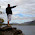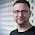## What is nools?

Nools is a rete based rules engine written entirely in javascript, with a target platform of node.js.
Nools was written for Pollenware to allow them to status offers in their market clearing events in a more dynamic way.

## Getting Started

To install nools run
`npm install nools`
Or
`git clone git@github.com:Pollenware/nools.git`
To run the tests
`make test`
To run the benchmarks
`make benchmarks`

## Terms

First lets start with a few terms that are used in nools.
• `rule` - A collection of constraints that must be satisfied for in order for a `action` to occur.
• `action` - The code that will execute when a all of a `rule`'s constraints have been satisfied.
• `fact` - An object/item inserted into a session that the `rule`'s constraints match against.
• `flow` - This is container for rules that will be executed, you can think of it as a rule book.
• `session` - This is an "instance" of a flow used to match facts. Sessions are obtained from a flow.

## Writing rules

Nools includes a parser to parse `nools` files into a `flow`.
Lets look at an example
```//Define our object classes, you can
//also declare these outside of the nools
//file by passing them into the compile method
define Fibonacci {
value:-1,
sequence:null
}
define Result {
value : -1
}

rule Recurse {
priority:1,
when {
//you can use not or or methods in here
not(f : Fibonacci f.sequence == 1);
//f1 is how you can reference the fact else where
f1 : Fibonacci f1.sequence != 1;
}
then {
assert(new Fibonacci({sequence : f1.sequence - 1}));
}
}

rule Bootstrap {
when {
f : Fibonacci f.value == -1 && (f.sequence == 1 || f.sequence == 2);
}
then{
modify(f, function(){
this.value = 1;
});
}
}

rule Calculate {
when {
f1 : Fibonacci f1.value != -1 {sequence : s1};
//here we define constraints along with a hash so you can reference sequence
//as s2 else where
f2 : Fibonacci f2.value != -1 && f2.sequence == s1 + 1 {sequence:s2};
f3 : Fibonacci f3.value == -1 && f3.sequence == s2 + 1;
r : Result
}
then {
modify(f3, function(){
this.value = r.result = f1.value + f2.value;
});
retract(f1);
}
}

```

### Defining Objects

In the above flow we define two classes `Fibonacci` and `Result` Both of these classes can be referenced by name throughout the file you can also access them by through the `flow` by calling `getDefined`.
In the nools language any valid `javascript` hash can be put inside of the define block, however if you define a `constructor` property then that will override the default constructor
For example we could change `Fibonacci` to to accept a sequence and value directly
```define Fibonacci{
constructor : function(sequence, value){
this.sequence = sequence || -1;
this.value = value || -1;
}
}
```
You could then use the new constructor in your rules
`var fib = new Fibonacci(10, 1);`

### Rule Definition

The rules `Recurse`, `Bootstrap` and `Calculate` use the nool rule syntax
```rule <name> {
when {<constraints>}
then {<action>}
}
```
Lets look at the `Recurse` rule a little closer.
```//Define a rule recurse
rule Recurse {
//Give it a priority. The priority is 1 which is above all
//other rules as the default priority/salience is 0
priority:1,
//Our LHS when block
when {
//you can use not or or methods in here
//give the fact an alias of f and we checking that there is
//NOT a Fibonacci object with a sequence of 1
not(f : Fibonacci f.sequence == 1);

//look for a Fibonacci object who's sequence does not equal 1
f1 : Fibonacci f1.sequence != 1;
}
then {
//assert a new fact into the engine
assert(new Fibonacci({sequence : f1.sequence - 1}));
}
}
```
Note In the when block each constraint must end with a `;`

#### But my action is async

The actions also support async actions through a `next(err?)` call.
```rule Recurse {
priority:1,
when {
not(f : Fibonacci f.sequence == 1);
f1 : Fibonacci f1.sequence != 1;
}
then {
process.nextTick(function(){
assert(new Fibonacci({sequence : f1.sequence - 1}));
next();
});
}
}
```
Note if next is called with an argument then it will be assumed to be an error and the `session` execution will stop and an error will be passed into the callback.

### Defining Helper Functions

Nools also allows the definition of helper functions, the function can be any valid javascript.
```function subtract(a, b){
return a, b;
}

function add(a, b){
return a + b;
}

function sum(){
var arr = Array.prototype.slice.call(arguments);
var sum = 0;
arr.forEach(function(a){sum += 0});
return sum;
}
```
You can reference the methods in your actions or within other defined functions.

## Working With Flows

The `flow` object is the container for all rules engines, a `flow` is created once and you can get multiple sessions from the flow.
To get a flow for the above fibonacci rules
```//compile the file into a flow
var flow = nools.compile(__dirname + "/fibonacci.nools");

//Get defined classes
var Fibonacci = flow.getDefined("Fibonacci"),
Result = flow.getDefined("Result");

```

### Sessions

The `flow` object is simply a conatainer for rules. To run the engine you need to obtain a `session`.
```var r1 = new Result(),
//Get a new session inserting a new Fibonacci object and a result to gather the
//final number
session1 = flow.getSession(new Fibonacci({sequence:10}), r1),
s1 = +(new Date());
//call match to execute the engine
session1.match(function(err){
if(err){
console.error(err);
}else{
console.log("%d [%dms]", r1.result, +(new Date()) - s1);
//cleanup the session
session1.dispose();
}
});
```

#### Session Events

Then `session` object also emits different events
• `fire` - emitted when a new rule is fired. The event is emitted with the name of the rule fired and associated facts.
• `assert` - emitted when a new fact is asserted
• `retract` - emitted when a fact is retracted(removed) from the session
• `modify` - emitted when a fact is modified

## Wrap Up

I hope this was a good introduction into nools for more information visit :

#### 23 comments:

1.Doug, this is cool stuff. Is this a port of Drools or a bottom up development effort? Any thoughts on performance or robustness at this point? I wonder if it would be possible to port some of the Drools tests over to test for completeness.

1.Thanks!

So I actually used drools to learn about rete but the implementation was inspired by both drools and ruleby but had to be heavily adapted to an evented platform. The benchmarks that are implemented are based off of ops5 benchmarks and are fully implemented so you will see more output than the corresponding drools implementation. I will always welcome more tests if you would like to contribute to the project you can fork and issue a pull request.

Performance is slightly slower than the drools but still performant. We are using it production at pollenware where thousands of facts are inserted into a session with no issues. However one thing to be aware of when implementing rules is to reduce the number of cross products that can be produced I found reducing the number of facts by inserting a "container" that is actually composed of what you need really helps as it reduces what could be multiple facts into one, however that is not always possible.

As for robustness we have not found a case yet where a lack of features has limited us.

I hope this helps!

2.Cool work!
I think it would be great if it had support for negation and aggregation too, as in Drools. Do you plan to add these capabilities?

1.Yes actually! Right now Im in the process of writing a from node implementation, then I plan on implementing aggregation nodes.

3.I agree really cool. Thanks for putting the effort into this.

4.I know that this question is trivial , but I have been trying for a few days to learn nools. Can u please explain in detail how each of the rules work in the Fibonacci example?

5.Really awesome package! Looking forward to further development and new features. I'm a newbie to rules engines and really appreciate the availability of this software. thank you

6.hi, cool stuff. Can nools be used in browser (not in node.js) ?

1.Yep! check out https://github.com/C2FO/nools#browser-support

7.Hi Doug,

Have I understood correctly that the amd compatibility is provided by browser/nools.js file in GitHub repo?

Thanks.

1.It is "https://github.com/C2FO/nools/blob/master/nools.js" that provides the support."

8.Hi Doug,

thanks for the tool, but there's one thing I don't understand of the syntax.

In this line:

f1 : Fibonacci f1.value != -1 {sequence : s1};

what s1 gets Bound to? Does it get bound to f1.sequence? So that means that I cannot user f1.sequence in the next line of my when?

Thanks.

1.In this scenario sequence would be bound to s1. While you could use f1.sequence directly, binding sequence to s1 reduces the terseness of the rest of the constraints since you do not have to type out f1.sequence every time.

9.I'm looking on using nools on the server side, is there a way to store/serialize session state?

1.This is not currently supported, however Im open to the idea Im not sure what the best approach would be since everything is determined by fact type. I would imagine if you wanted to do this a mechanism to serialize and deserialize custom fact types would be needed.

10.This is really interesting, I have a naive question: How is nools relate to expert.js (https://github.com/L3V3L9/expert) Do they solve the same sort of problems, or does nools focus on the chaining and expert focuses more on the semantic meaning and relationships?

Cheers
Rob

1.After a cursory look through the README it looks like expert focuses more on providing a list of facts and you as questions in order to gain knowledge of the the domain. Where nools is a rule engine that focuses on providing a list of facts and providing a list of answers to predefined questions. Hope this helps.

11.Hi Doug,
I just started using Nools with Node.js. I want to know if I can decouple it from the application to provide stateless service, like Drools server.
Also, I want to know, how to access the class members outside the nools file.

Thanks in Advance.

1.For the first part I have not considered that possibility but I am always accepting pull requests :)

For the second question, if I understand your question you can access them by using flow.getDefined("myDefined")...of course replacing myDefined with the class name specified when defining the class.

12.Hi Doug,
I want to know if we can return a value from the rules action,like,
define sum{
a : 0,
b : 0,
c : 0,
constructor : function(x,y){
this.a = x;
this.b =y;
}
}
rule "one"{
when {
s : sum s.a >3 && s.b>5;
}
then {
c = a+b;
return c;
}
}

And also, can we use the helper functions in rule actions? If so, where is the value returned to?
Thanks in Advance.

13.Hi Doug,
Is it possible to define rules dynamically completely at runtime ? If so, how? I am using node.js and the rules are in oracle database. I am storing it in the DB because, I want to provide the service of CRUD for rules.

14.Hi Doug,
There is a drools-server war which can be deployed to execute KnowledgeBases remotely for any sort of client application and any technology that can use HTTP, through a REST interface can use it. So is it possible to use drools execution server module from node.js?

15.Hi Doug,
I couldn't figure out, to where the helper function values are returned and how to capture it. I want to return an array and access its values. Can u please help me with it?

Thanks in Advance.